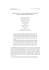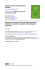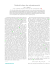# Extended phase diagram of the Lorenz model

## Transcription

Extended phase diagram of the Lorenz model
```Extended phase diagram of the Lorenz model
Holger R. Dullin1,2 , Sven Schmidt2 , Peter H. Richter2 , Siegfried K. Grossmann3
1
Mathematical Sciences Loughborough University,
LE11 3TU, Loughborough, UK
2
Institut fuer Theoretische Physik der Universität Bremen,
D-28334 Bremen, Germany
3
Fachbereich Physik der Philipps-Universität,
Renthof 6, D-35032 Marburg, Germany
(Dated: April 12, 2005)
Abstract
The parameter dependence of the various attractive solutions of the three variable nonlinear
Lorenz model equations for thermal convection in Rayleigh-Bénard flow is studied. Its bifurcation
structure has commonly been investigated as a function of r, the normalized Rayleigh number, at
fixed Prandtl number σ. The present work extends the analysis to the entire (r, σ) parameter plane.
An onion like periodic pattern is found which is due to the alternating stability of symmetric and
non-symmetric periodic orbits. This periodic pattern is explained by considering non-trivial limits
of large r and σ. In addition to the limit which was previously analyzed by Sparrow, we identify
two more distinct asymptotic regimes in which either σ/r or σ 2 /r is constant. In both limits the
dynamics is approximately described by Airy functions whence the periodicity in parameter space
can be calculated analytically. Furthermore, some observations about sequences of bifurcations
and coexistence of attractors, periodic as well as chaotic, are reported.
1
I.
INTRODUCTION
The nonlinear coupled set of three ODEs
ẋ = −σx + σy ,
ẏ = rx − y − xz ,
ż = −bz + xy ,
(1)
was first derived by Berry Saltzman and Edward Lorenz [1, 2] as a model for thermal
convection between two plates perpendicular to the direction of the earth’s gravitational
force. It has three control parameters. First, there is the normalized temperature difference
r = ∆T /∆Tc = Ra/Rac between the hotter lower plate and the colder upper one. ∆Tc is the
temperature difference at the onset of convection. Ra = gαp L3 ∆T /νκ, the Rayleigh number,
is the dimensionless temperature difference. g denotes the gravitational acceleration, αp the
isobaric thermal expansion coefficient, L the distance between the plates in upward direction,
and ν, κ characterize the kinematic viscosity and thermal diffusivity, respectively.
Second, there is the Prandtl number σ = ν/κ, describing the ratio of the two molecular
time scales τν = L2 /ν and τκ = L2 /κ for momentum and energy transport in the system.
σ also measures the ratio of the widths λu and λT of the kinematic and the thermal boundary
layers. Large Prandtl numbers σ indicate a broad kinematic boundary layer containing
a small thermal one, fast molecular momentum exchange and small convective Reynolds
numbers. Small Prandtl numbers characterize fast molecular thermal exchange, a broad
thermal boundary layer containing a thinner kinematic one and larger convective Reynolds
numbers. Typical values for σ are 0.7 in air (at room temperature), N2 , O2 , He, and other
gases, about 7 in water (room temperature), 600 through 3000 in typical organic liquids as
e.g. dipropylene glycol (600), or σ ≈ 7250 in glycerine. Small Prandtl numbers are realized
in mercury (σ ≈ 0.025) or liquid sodium with σ ≈ 0.005.
The third parameter in eqs. (1) is the coupling strength b, originating from the nonlin~
earity (~u · ∇)T
of the Boussinesq equation. It is of order 1 and conventionally chosen as
b = 8/3; we adopt this value here.
The variables x(t), y(t), and z(t) describe, respectively, the amplitude of the velocity
mode (the only one left in the model), the amplitude of the corresponding temperature
mode, and the amplitude of the mode measuring the nondimensional heat flux Nu, the
Nusselt number. x, y characterize the roll pattern, the heat flux is
Nu = 1 + 2z/r .
2
(2)
For 0 ≤ r ≤ 1 there exists one stable fixed point P0 (x0 = y0 = z0 = 0), with Nu = 1,
corresponding to pure molecular heat conduction. For r exceeding 1, convective rolls develop,
described by two stable fixed points
p
p
P± = (x± , y± , z± ) = ± b(r − 1), ± b(r − 1), r − 1
(3)
hence Nu = 3 − 2/r. The trajectories in phase space Γ = {x, y, z} are spirals towards these
fixed points. Stability is lost for
r > rc = σ
σ+b+3
.
σ−b−1
(4)
For the canonical choice σ = 10 it is rc = 24.7368.... At such large r the three mode approximation for the PDEs describing thermal convection has of course ceased to be physically
realistic, but mathematically the model now starts to show its most fascinating properties,
because a strange attractor develops for r > rc . With further increasing r, windows of regular behavior emerge out of chaos where stable periodic orbits exist. These stability windows
tend to become broader and broader until for sufficiently large r chaos seems to disappear
and one type of stable periodic solution takes over.
Of the numerous studies of the Lorenz model we cite as examples the monograph  and
the more recent survey in Jackson’s book . To the best of our knowledge, the parameter
dependence of the Lorenz model has mainly been analyzed with respect to its control parameter r, the external forcing, and sometimes with varying b (as in the work  which is
cited in ). The Prandtl number σ has usually been fixed to its canonical value σ = 10, and
as a rule, b has been taken as 8/3. The limit of large r at arbitrary fixed σ was thoroughly
studied by Robbins  and Sparrow .
In the present paper we study the parameter range where both r and σ become large.
The scheme of bifurcations is then a very different one. With σ as large as 60 (Fig. 1) one
is just beginning to see a structure that unfolds on much larger scales (Fig. 2). There is
ongoing repetition of a few basic features, but these are different from the structure at low σ.
√
The line σs = br divides these two regimes as we shall show. Our theoretical explanation
of these findings focuses on a backbone structure of the dominant stable periodic attractors,
but orbits with arbitrarily high periods, and chaos, are also observed as part of the pattern.
An important feature of the Lorenz equations is their invariance with respect to the
reflection
Σ:
x, y 7→ −x, −y
3
and z 7→ z .
(5)
Evidently the z-axis is a Σ-invariant line. For r > 1 it is also the stable manifold of the
fixed point P0 . This characteristic symmetry property with respect to Σ has implications
on the nature of the period doubling scenarios which occur over and over again as the
parameters r, σ are varied: they do not follow the familiar scheme of the paradigmatic
logistic iteration x 7→ ax(1 − x) but rather the somewhat more intricate scheme of the
cubic iteration x 7→ f (x) = (1 − c)x + cx3 . This cubic mapping possesses the symmetry
f = Σ̃−1 f Σ̃, with Σ̃ : x 7→ −x, and may be viewed as one building block for understanding
the transition to chaos in the Lorenz system, see Hao et al. [7, 8] for a detailed analysis of
this aspect.
The motivation of the present work was the simple question why the meteorologist Lorenz
would have chosen the Prandtl number σ = 10, which is adequate for water but less so for
air with its order of magnitude σ ≈ 1. The reader will easily find that taking σ this small,
he will no longer find chaotic solutions. This is confirmed by a glance on eq. (4): with σ = 1,
the critical parameter rc = −5/2 lies in the unphysical range r < 0. If one chooses, instead,
some σ > b + 1, implying rc > 0, the window structure in the r-dependence is similar to
the case σ = 10 although the details may be nontrivially different. But one hardly sees how
these differences in the r-behavior for some discrete σ-values are connected. This motivated
us to vary σ in sufficiently small steps and study the 2-dimensional (r, σ)-space of control
parameters in a larger domain than has hitherto been done. However, quite apart from such
a physical motivation, the mathematical complexity of this innocent looking set of equations
warrants an investigation of their asymptotic behavior where it has not yet been explored.
In Section II we present our parameter survey (phase diagrams) and discuss its main
features. Then, in Section III the nature of two kinds of periodic orbits is described whose
stability regions define the dominant regular features of the large scale phase diagram; an
analytic derivation of these observations is given in Section IV. More complex aspects of the
Lorenz dynamics such as bifurcations of periodic orbits, chaos, and coexistence of attractors,
are discussed in Section V. We point out regularities, and similarities to other well known
systems, but do not give detailed explanations of these features.
4
II.
TWO-PARAMETER ANALYSIS
A glance at Figs. 1 and 2 gives an impression of the bifurcation scheme on large and very
large scales of r (abscissa) and σ. The parameter b, as mentioned already, is kept at its
standard value 8/3. Colors indicate the existence of attractors of a certain type, which does
not preclude the coexistence of others. More precisely, the figures are parameter scans where
each point (r, σ) is colored according to which type of attractor is approached from a fixed
initial point (x0 , y0 , z0 ). Such pictures are called final state diagrams, or phase diagrams.
The simplest attractors are the fixed points P± , see (3). Their stability is lost along the
curve r = rc (σ), see (4), or rather by its inverse function σ = σc (r) with
σc (r) =
r b + 3 1p 2
−
±
r − 2(3b + 5)r + (b + 3)2 .
2
2
2
(6)
For large r, the upper and lower asymptotes σu (r) and σℓ (r) are, respectively,
σu = r − 2(b + 2) and σℓ = b + 1 .
(7)
The next order correction is ∓(b + 1)(2b + 4)/r, describing the approach of the upper asymptote from below and the lower one from above.
The (r, σ)-region where the points P± are stable according to this linear analysis is colored
black, the rest is left white, before the other colors are added in Figs. 1 and 2. These colors
code for the periods of attractors which are determined by the procedure described below:
yellow for orbits of period 1, red for period 2, green for period 4, blue for period 8, grey for
period 3, olive for period 6.
For each (r, σ) on a grid of 610 × 620 points, an orbit was started with initial conditions
(x0 , y0 , z0 ) = (0.001, 0.001, r − 1). A transient regime of 80 000 iterations was allowed to
elapse before it was assumed that an attractor was reached. The trajectory was then analyzed in terms of its intersections with the (x, y)-plane at z = r − 1 which is an appropriate
Poincaré surface of section. The period p of a periodic orbit is defined as half the number
of intersection points of the orbit with that plane (in one half the trajectory intersects from
above, in the other half from below). Fig. 3 gives two examples for illustration. To determine the period we used a rather simple scheme. A disk of radius 10−3 was placed around
each point of intersection unless it fell in a disk already generated. When the number of
patches so obtained remained finite, like in the left part of Fig. 3, the orbit was assumed to
5
FIG. 1: (r, σ)-phase diagram in the range 0 ≤ r ≤ 409 and 0 ≤ σ ≤ 60. Colors indicate the type
of attractor reached from the initial condition (x0 , y0 , z0 ) = (0.001, 0.001, r − 1): fixed points P±
(black), orbits of period 2 (red), period 4 (green), period 8 (blue), chaotic attractors (white). This
√
picture is a zoom into the lower left corner of Fig. 2. The line σs := br in color magenta marks
a transition range between different global structures for smaller or larger σ. Further details are
discussed in the text, Section V A.
be periodic. When, on the other hand, the number of disks seemed to grow indefinitely, like
in the right part of the figure, the orbit was considered to be chaotic. In practice we waited
for more than 100 intersections, but only periods up to 8 form clearly identifiable bands on
the scales of the figures. When a periodic orbit was detected, the color of the grid point
(r, σ) was overwritten with the color of the code given above. Otherwise the original black
6
FIG. 2: The parameter ranges are 0 ≤ r, σ ≤ 2000. The color code is the same as in Fig. 1.
Yellow bands indicate stability of orbits with period 1 (not present in the zoomed lower left corner,
cf. Fig. 1). The dots in the red and yellow bands along the line σ = 0.75 r are, from bottom left to
top right, S1 , A1 , S2 , A2 , . . . , defined according to (44) for rN (m = 0.75). With their upper parts,
the bands penetrate well into the range above σu = r − 28/3, cf. (7) (the line can be identified as
the border to the black region through the bands), indicating coexistence of periodic orbits with
the stable fixed points P± .
7
(a1)
(a2)
(b1)
(b2)
FIG. 3: (a1): (y, z)-projection of an asymmetric attractor of period 3 (abscissa y, ordinate z).
r = 1744, σ = 110, initial conditions x0 = y0 = 10−5 , z0 = r − 1. The fat red points are the
fixed points P± . (a2): The same for a chaotic attractor; r = 1744.9, σ = 115.2. (b1) and (b2):
Corresponding Poincaré sections with the (x, y)-plane at z = r − 1; intersection points are blue if
from below, red if from above (abscissa x, ordinate y).
or white color was left unchanged. Hence chaotic attractors appear as black or white points.
The method produces two artifacts. First, it cannot identify the fixed point attractors P± ;
hence, in the black region it does not distinguish between fixed points and chaotic attractors.
Second, when the relaxation towards an attractor is very slow, the 80 000 transient iterations
may not be sufficient to reach it, and the method produces more than 100 patches. Hence
this situation is not distinguished from chaos. It is observed in connection with critical
slowing down in the neighborhood of a bifurcation of the attractor. Examples are the white
bands in the red regions at bottom and top of Fig. 1, the narrow seams between red and
green regions, and the lines inside the two green bands in the chaotic range. The nature of
the corresponding bifurcations will be elucidated in the following section.
Since only one initial condition is used, the procedure does not determine whether different initial conditions would lead to different attractors for the chosen parameters (r, σ).
8
The question of coexistence of attractors will be addressed later, in Sec. V B. At this point
we can assert already that where a colored band extends into the black region, like in the
lower right and upper left parts of Fig. 1, the corresponding periodic orbit coexists with the
fixed points P± .
The arrangement of bands in Fig. 1 exhibits a qualitative structural symmetry with
respect to a line which has been added in magenta color. This line, mathematically defined
√
as σs = br, separates a large-σ structure from a kind of mirror image in the low-σ part of
the phase diagram. It is conspicuous how every band below σs (r) seems to have a counterpart
on the other, upper side. But, as a glance on Fig. 2 shows, this “symmetry” disappears on
a larger scale: there is no repetitive structure in the low-σ region, in contrast to the large-σ
range.
No orbits of period 1 (yellow bands) are observed in the (r, σ)-range covered in Fig. 1.
However, in Fig. 2 which covers a much larger (r, σ)-range, an onion like repetitive pattern
of yellow (period 1) and red (period 2) bands dominates in the upper part. These bands
are separated from each other by chaotic regions which also contain small bands of higher
periods. The bands (above σs ) have an hyperbolic shape whose upper branches seem to have
asymptotic slopes of approximately 1.3 while the lower branches will be shown in Section IV
√
to have a limiting behavior σ ∝ br like the “bottom line” σs (r). The upper branches
penetrate significantly into the region σ > σu where the fixed points P± are stable, see
eq. (7). This means there is coexistence of fixed point attractors and periodic attractors. To
generate Fig. 2 the initial conditions were x0 = y0 = 10−5 and z = r + 1000. Choosing the
initial conditions as in Fig. 1, the corresponding picture (not shown here) looks different in
that the greater part of the white bands of chaos is replaced by colors yellow or red. This
reflects the coexistence of chaotic and periodic attractors.
The bands have infinite vertical slopes along the line σv (r) ≈ 0.75r. On this line, we
have marked points S1 , A1 , S2 , A2 , S3 , ... in the red (period 2) and yellow (period 1) bands,
respectively. The names of these points will also be taken to denote the corresponding bands
because the attractors of a given band are topologically identical. Their nature will first
be described phenomenologically in the subsequent section, where we shall also see how it
changes from one band to the next. An analytic explanation of the findings will then be
given in Section IV.
9
III.
ATTRACTIVE PERIODIC ORBITS
In this section we explore the nature of the dominant periodic attractors, i. e., of the orbits
with periods 1 or 2, by means of numerical and graphical investigation. Their bifurcations
into orbits of higher periods, as well as into strange attractors, will be discussed in Section V.
To begin with, consider the attractors corresponding to the (r, σ)-values of A3 in Fig. 4
and of S4 in Fig. 5, in the three standard projections. Comparison with the S1 , A1 , S2 , and
A2 attractors (see Figs. 7 and 16) shows that on the scales used, −0.4r < x, y, z − r < 0.4r,
all An attractors look alike, and so do the orbits of Sn . Furthermore, the attractor of the
parameter point Sn (for given n) looks like a combination of the asymmetric orbits of An
and its Σ-mirror image ΣAn .
The time course of the variables x, y, and z is plotted in Fig. 6, both for the A3 attractor
(top) and the S4 one (bottom).
The combined picture shows the typical behavior of a relaxation oscillator. Two different
regimes can clearly be distinguished: First, the variable z decreases slowly from an upper
value r(1 + ρ̂) to a lower value r(1 − ρ̂), with ρ̂ ≈ 0.35, while x and y are virtually zero.
Then, in a very fast process, x and y start out on a large excursion with amplitude r ρ̂, while
z jumps back to its upper value. The (y, z)-projection exhibits an almost perfect half circle;
the (x, y)-projection shows that during the excursion, x lags somewhat behind y.
10
FIG. 4: Stable asymmetric periodic orbit corresponding to the parameters (r, σ) = (1322, 992)
of the point A3 in Fig. 2. From left to right, the attractor is shown in (x, y), (x, z), and (y, z)
projection (the abscissa is always mentioned as first coordinate, the ordinate as second). The dots
mark the projections of the fixed points P± , see (3). The arrows indicate the direction of flow;
the decrease of z takes place very close to the z-axis. The attractor coexists with its Σ-partner
which is obtained by reflection of x and y. Together these two attractors look like the symmetric
attractor in Fig. 5. The scales are −530 < x, y, z − r < 530.
FIG. 5: Stable symmetric periodic orbit corresponding to (r, σ) = (1552, 1164), the point S4 in
Fig. 2. Same projections as in Fig. 4. The scales are −620 < x, y, z − r < 620.
11
FIG. 6: Time development of x, y, and z within one unit of time t (abscissa), for the attractors of A3
(top) and of S4 (bottom). The upper parts show the z-coordinates, the lower parts x and y which
are nearly indistinguishable on this time scale. The vertical scale is −0.4 r < x, y, z − r < 0.4 r.
FIG. 7: (x, y)-projections of (from left to right) the attractors of S1 , A1 , S2 . In the case of A1 the
two asymmetric orbits are shown in different color. The insert in the left picture is a blow-up of the
neighborhood of the origin. The (r, σ) values are S1 = (172,130), A1 = (402,302), S2 = (632,474);
the scales are −0.4 r < x, y < 0.4 r.
12
FIG. 8: Successive blow-ups of the box in the middle picture of Fig. 7. The sequence demonstrates
that the two orbits of A1 do not reach the origin; rather each of them winds once around the z-axis.
From Figs. 4-6 it is not obvious what happens during the slow phase where the orbits
move down along the z-axis. This question is clarified by the sequence of (x, y)-projections
in Figs. 7 and 8. The individual pictures in Fig. 7 show the attractors of S1 , A1 , S2 ; the other
projections would be similar to those in Figs. 4 and 5. Taken together, the pictures make it
clear that the orbits wind around the z-axis on spirals with decreasing R2 = x2 +y 2 . Coming
from large values of R, near the maximum value of z, they enter a close neighborhood of
the z-axis when they pass a point where y = 0. After a quarter turn in clockwise direction
(looking down the z-axis) there comes a point where x = 0, at smaller values of z and R.
What happens next depends on the index n of Sn or An . In the case of S1 the orbit quickly
leaves the (x, y)-origin, cf. the inset in the left part of Fig. 7; it performs a large loop and
returns to the (x, y)-origin region from the other side, but again near the maximum of z.
All in all it encircles the z-axis once, its winding number W with respect to the z-axis
being equal to the number N = 1 of zeros in y (and correspondingly in x) upon each close
encounter. In the case of A1 , the orbit stays longer near the z-axis, with a second zero of y
and also of x, each with smaller values of R (and z); this is illustrated by the sequence of
zooms in Fig. 8. Its winding number with respect to the z-axis is also W = 1, but now there
are two of these orbits, shown in different colors. To keep track of the winding number W
for increasing band label n, we may focus on the number N of zeros of y (or x) upon close
encounter with the z-axis. Detailed observation shows that this number is
W (Sn ) = N(Sn ) = 2n − 1
and
W (An ) = 21 N(An ) = n.
(8)
Fig. 9 shows a logarithmic plot of |x| and |y| for A3 (N = 6, W = 3) and S4 (N = 7, W = 7).
We observe three different regimes of time dependence. Soon after z reaches its peak value,
13
at a time t0 , the first zeros of y and x occur, visible as logarithmic singularities. The first
regime of oscillatory exponential decay of x and y ends at a time t1 . In the second phase
there are no further x- or y-zeros, but the z-decay continues with deceleration up to a time
t2 where the distance R of the orbit from the z-axis reaches a minimum. The third regime
is one of explosive, i. e., faster than exponential increase to the original values of x, y and z.
The spiraling behavior in the first regime seems reminiscent of “Shilnikov Chaos” .
However, in this case all the relevant dynamics stays far away from the fixed point. The
invariant line x = y = 0 is part of the stable manifold of the origin, but this is not important
for our arguments. The main ingredient is that it is attractive for z > r (i. e. up to time
t2 ) and repulsive for 0 < z < r. Long before the orbit gets close to the origin it leaves the
z-axis along its unstable eigenvector, with x − y ≈ 0.
FIG. 9: Time development of log |x| (red), log |y| (blue), and z (green) within 0.4 time units, for
the attractors of A3 (top) and of S4 (bottom). The vertical scales are the same as in Fig. 6. x and y
are plotted as 30 log |x, y| + 600. Thus the x- and y-zeros appear as logarithmic singularities.
The global structure of the (r, σ) parameter space, as exhibited in Fig. 2, is obviously
14
related to the existence and stability of such orbits, with winding numbers W (An ), W (Sn )
increasing as r and σ (and thereby n) grow. The observations to be explained in the
subsequent theoretical section are the following:
1. Repetition of bands: Along the line σ = mr with a fixed ratio m, there exists a period q
in r (and consequently mq in σ) such that if an attractor of type An (or Sn ) exists
for values (rn , σn ), then a corresponding attractor of type An+N (or Sn+N ) exists for
(rn + Nq, σn + Nmq).
2. m-dependence of r-periods q: From the shape of the bands it may be inferred that
q(m) has a minimum near m = 0.75. This means, the periodic repetition of the bands
is fastest (in r) along the line σ = 0.75r.
3. Size scaling of dominant orbits with r: The size of these orbits, i. e., the radius ρ̂
of their (y, z)-projections measured in units of r, is independent of r: ρ̂ ≈ const for
fixed m.
Thus far we have not addressed the question of how ρ̂ depends on m, so let us see next what
theory has to tell; the result is presented in Fig. 11 where ρ = ρ̂/m is plotted as a function
of m. Of course, the above statements only hold for large r and σ, in practice for r & 100
√
and σ in the bands above σs = br.
IV.
ANALYSIS OF THE PARAMETER SPACE STRUCTURE
We begin with a discussion of appropriate scalings of the entire set of Lorenz equations (1)
when both parameters r and σ become large. Their asymptotic behavior with r → ∞ depends on how σ is assumed to change simultaneously. Robbins in  and also Sparrow 
considered the case σ = const. Here we study the behavior of the Lorenz equations with
Prandtl numbers increasing as σ ∝ r s , with some fixed exponent s > 0 to guarantee increasing σ, but s ≤ 1 for σ to stay below the upper stability border σu (r) = r − 28/3.
If r and σ become very large, the motion in x and y is expected to become very fast
because d/dt in the equations of motion becomes large. Thus we introduce a rescaled,
stretched time τ by defining dτ = ε−1 dt with a scaling factor ε−1 ∝ r e , e > 0.
Let us now also rescale the variables x and y which measure, respectively, the amplitudes
of the role velocity and temperature. As for the rescaling of z a glance on the second Lorenz
15
equation shows that y is driven by (r − z). This fact is reflected in the amplitudes z of
the numerical trajectories, or in the behavior of the fixed points P± , both as functions of r.
Thus we define
x = αξ,
y = βη,
z − r = γζ.
(9)
The Lorenz equations then read, with ξ ′ = dξ/dτ etc.,
β
ξ ′ = −εσξ + εσ η,
α
α
η ′ = −εη − εγ ζξ,
β
r
αβ
ζ ′ = −εb(ζ + ) + ε ξη.
γ
γ
(10)
The freedom of choosing scaling parameters may now be used to normalize to unity the
coefficients of the driving term in the first equation, and of the two coupling terms ∝ ζξ and
εσβ = α,
εγα = β,
εαβ = γ.
(11)
These equations allow us to express α, β, and γ in terms of σ and ε. We find first ε2 α2 = 1,
thus α = ±ε−1 , then β = ±ε−2 σ −1 , and finally γ = ε−2 σ −1 . The ± signs may be interpreted
as corresponding to the symmetry Σ which is clearly preserved under the rescaling. We can
therefore restrict ourselves to the plus sign and have
α = ε−1 ∝ r e ,
β = γ = ε−2 σ −1 ∝ r 2e−s .
(12)
The asymptotic behavior will depend on how the exponent e compares to the other exponent 2e − s. There appear to be three distinct possibilities. One is that β and γ grow faster
than α, i. e., 2e − s > e or e > s. The second is that all three variables x, y, z scale with
the same factor, 2e − s = e or e = s. The third possibility e < s may be excluded with the
argument that then the relaxation term −εσξ in the ξ-equation would diverge.
A representative case of the first possibility is s = 0 and e = 21 , meaning σ = const and
√
ε ∝ 1/ r → 0. This was the choice of Robbins  and Sparrow . All dissipative terms
vanish in this limit, and the equations reduce to
ξ′ = η ,
η ′ = −ζξ ,
ζ ′ = ξη ,
√
(σ = const, ε = 1/ r).
(13)
This system has the two integrals A = 21 ξ 2 − ζ and B 2 = η 2 + ζ 2; it is equivalent to the
equations of a pendulum with length B and energy A. The values of the integrals A and B
have to be determined from the ε-correction to (13), i. e., by considering their slow dynamics.
In [3, 6] it was shown how this can be done in terms of elliptic integrals. In that sense, this
case has been solved.
16
Let us therefore concentrate on the second possibility e = s. Then εσ is independent of r,
and we can take εσ = 1. All scaling factors can now be expressed in terms of the Prandtl
number σ. The term −εη in the second Lorenz equation may be neglected as vanishingly
small in comparison to with ζξ. Hence the rescaled eqs. (10) become
ξ ′ = −ξ + η,
η ′ = −ζξ,
ζ ′ = ξη −
br
b
ζ − 2,
σ
σ
(e = s and σ = r s ) .
(14)
But what about the two last terms in the ζ-equation? Their order of magnitude is br −s and
br 1−2s , respectively. Again, there are three possibilities:
Case A. With the choice 1 − 2s = 0, or s = 1/2, the second term will tend to a constant and
√
dominate the decreasing first term. We can write σ = w br with some constant w. The set
of equations becomes
ξ ′ = −ξ + η,
η ′ = −ζξ,
ζ ′ = ξη − w −2
(e = s =
1
2
√
and σ = w br) .
(15)
When s varies between 1/2 and 1, the same equations apply because the last term in the
ζ-equation of (14) dominates the second. Note that no comparison can be made between
these two terms and ξη.
Case B. When s = 1, the two last terms in the ζ-equation are of the same order of magnitude r −1 . We write σ = mr and obtain the set of equations
′
ξ = −ξ + η,
′
η = −ζξ,
1
b ζ+
ζ = ξη −
mr
m
′
(e = s = 1 and σ = mr) . (16)
Case C. When s > 1, σ grows faster than r, so we leave the range where σ < σu (r). Formally
it is found that both terms besides ξη in the ζ-equation may be neglected, but in contrast
to eqs. (13) there remains a dissipative term in the ξ-equation. The constant of motion
B 2 = η 2 + ζ 2 constrains the solution to a cylinder. On this cylinder the equation reduces to
the damped pendulum, and therefore almost all initial conditions decay towards the stable
equilibrium on the respective cylinder.
We thus have to discuss the asymptotic regimes A and B, and do this separately. To our
knowledge, the two sets of eqs. (15) and (16) have not been analyzed so far. As we shall
see, their solutions lead to an understanding of the phase space structure displayed in Fig. 2
and of the numerical findings in Section III.
17
A.
√
The limit of large r with σ = w br
We start with the simpler case of the asymptotic Lorenz equations, the set of the rescaled
eqs. (15). Of all the complex dynamics that it contains, we only want to describe periodic
orbits of the simplest kind. From our numerical studies we anticipate that these orbits have
two clearly distinct phases. There is slow motion down along the z-axis, and a fast return
to the original large value of z via a large loop away from the z-axis.
The fast motion is readily described when we neglect the constant term in the ζ-equation
of (15). Then, combining the equations for η and ζ, we obtain ηdη = −ζdζ which after
integration gives the circles
η 2 + ζ 2 = ρ2
(17)
with as yet undetermined radius ρ. A convenient parametrization of these circles is found
with a rescaling of time, defining s as an arc length via ds = ξ(τ )dτ . The equations for η(s)
and ζ(s) are thereby linearized, with solutions
η(s) = ρ sin s,
ζ(s) = −ρ cos s .
(18)
This explains the observation that ζ oscillates between two equal values of different sign,
namely ±ρ.
The equation for ξ,
dξ
= −(ξ − η)
dτ
dξ
η(s) =− 1−
ds
ξ(s)
or
(19)
does not appear to be analytically solvable, but as long as ξ is not too small, it describes a
relaxation of ξ(s) towards η(s). Hence, with a delay in τ of the order of 1, ξ follows η on its
excursion to values of the order of ρ.
Consider now the slow motion. It is given by the exact dynamics on the stable manifold
ξ = η = 0 of the fixed point (0, 0, 0). The solution with initial point ζ(0) = ρ is ζ(τ ) = ρ −
τ /w 2 and reaches the lower value −ρ at time T = 2ρw 2 . Linearizing the set of equations (15)
around this motion, we obtain
ξ ′ = −ξ + η ,
The τ -dependent eigenvalues are − 21 ±
η ′ = −(ρ − τ /w 2 )ξ .
p
∆(τ ) with discriminant ∆ =
(20)
1
4
− ρ + τ /w 2 . Three
τ -regimes may be identified. Assuming ρ > 1/4, the discriminant is initially negative; the
18
motion is oscillatory and decaying according to e−τ /2 . This regime ends at the time τ1
defined by ∆(τ1 ) = 0, or τ1 = w 2 (ρ − 41 ). In the second regime, the motion continues to be
decaying but is no longer oscillatory; this is the case between τ1 and τ2 = w 2 ρ = T /2 where
ζ(τ2 ) = 0. The third regime, between τ2 and T , sees ξ and η increasing at a growing rate.
To proceed with the (ξ, η)-equations, we introduce a new variable X by separating the
exponentially decaying part from ξ. With ξ(τ ) = e−τ /2 X(τ ), the two linear equations of
first order are transformed into
X ′′ − ∆(τ )X = 0 ,
or
X̃ ′′ −
τ̃
X̃ = 0
w2
(21)
where τ̃ = τ − τ1 , and X̃(τ̃ ) = X(τ − τ1 ) lives on the interval −τ1 < τ̃ < T − τ1 . This
equation is the well known Airy equation. Its general solution is a superposition of the
two Airy functions Ai(z) and Bi(z) where z = w −2/3 τ̃ . Both solutions oscillate for z < 0.
For z > 0 the solution Ai decays whereas Bi grows exponentially. We are interested in the
solution X̃(τ̃ ) = Bi(z), or
ξ(τ̃ ) = e−τ1 /2 e−τ̃ /2 Bi(w −2/3 τ̃ ) .
(22)
with −τ1 < τ̃ < T − τ1 . It may be checked that η(τ̃ ) = e−τ /2 Y (τ ) is governed by the same
Airy equation and thus, up to a phase shift, has the same solution. An example of the
function Bi is shown in the left part of Fig. 10, where the parameters are those of S4 (the
plots are made on the basis of eqs. (40)-(42)). The corresponding ξ(τ̃ ) is shown in the right
part of the picture, in logarithmic representation.
From here it is straightforward to determine the value of ρ, and the periodicity of the
pattern of bands.
We know from the analysis of the outer part of the attractor, see (18), that the solution
starts, for τ = 0 or τ̃ = −τ1 , at about the same values ξ and η at which it ends for τ = T :
ξ(0) = ξ(T ) .
(23)
Using the asymptotic behavior of Bi for large negative and positive arguments,
1
cos 23 |z|3/2 + π4 ,
1/4
π|z|
1
Bi(|z|) ≈ √
exp{ 32 |z|3/2 } ,
π|z|1/4
Bi(−|z|) ≈ √
(24)
and the fact that the exponential terms dominate the algebraic factors by orders of magnitude, we simply equate the exponents on the two sides of (23). For τ̃ = −τ1 eq. (22) gives
19
ξ(0) = O(1) (zero exponent); for τ̃ = T − τ1 , we add up the exponents in eqs. (22) and (24),
with z = w −2/3 (T − τ1 ), and obtain
3/2
τ1 T − τ1 2 −2/3
1
21
0=− −
(T − τ1 ) = − T +
+ w
(T − τ1 )3/2 .
2
2
3
2
3w
(25)
Inserting T = 2ρw 2 and τ1 = w 2 (ρ − 41 ) we obtain
144ρ2 = (1 + 4ρ)3 .
(26)
The relevant solution is ρ = 1.35... =: ρ0 .
To determine the values w = wN where the orbits have a certain number N of ξ- and
η-zeros at each close encounter with the z-axis, we use the asymptotic behavior of the Airy
function Bi(−|z|) as given in (24). Consider first the symmetric orbits Sn as they develop
from time τ̃ = −τ1 to T − τ1 . The coordinates ξ and η come in from large values (positive
or negative) and go out to large values of the opposite sign. This means the phase shift
between ξ(τ̃ = −τ1 ) and ξ(τ̃ = T − τ1 ) is π; the cosine performs an odd number of half
oscillations. For the asymmetric orbits An , on the other hand, the phase shift is a multiple
of 2π. Taken together, this leads to the “quantum condition”
2 1 3/2
τ
3w 1
+
π
4
= Nπ ,
(27)
where N = 1 corresponds to the first symmetric and N = 2 to the first asymmetric band.
With ρ = ρ0 we get
√
wN = 1.02 4N − 1 ,
N = 1, 2, ... .
(28)
To wrap it up, the results for this regime of large r are the following. The N-th band
√
is centered around the line σN = wN br; the size of the corresponding attracting periodic
orbits in the original variables (x, y, z) is ρ0 σN . But note this is only asymptotically true
and can hardly be seen in the diagram of Fig. 2 except in the lower right corner where
√
the S1 -band is just bending over to follow the r behavior. r = 2000 gives the prediction
√
σ1 = 74.5 3 = 129 which lies at the lower border of the S1 -band. The bands corresponding
to higher values of N have not yet entered this asymptotic regime.
B.
The limit of large r with σ = mr
Let us now study the set of equations (16)
ξ ′ = −ξ + η,
η ′ = −ζξ,
20
ζ ′ = ξη −
b 1
ζ+
,
mr
m
where m = σ/r is the constant parameter. Much of the analysis is similar as in the previous
subsection, but the explicit occurrence of r in the equations adds complications. The general
strategy is the same. For the outer, large ξ and η parts of the solution we neglect the term
∝ r −1 in the ζ-equation and get identical results as in (17)-(19).
The slow motion along the z-axis is more complicated even though the ζ-equation, neglecting the ξη-term, decouples again from the rest: its solution is no longer linear in τ .
With the initial condition ζ(0) = ρ the approximate ζ ′ -equation is solved by
ζ(τ ) = (ρ +
1
)e−(b/mr)τ
m
−
1
,
m
(29)
and the time T needed to evolve from ρ to −ρ is
T =
1 + mρ
mr
log
.
b
1 − mρ
(30)
Using this slow exponential decrease of ζ (with the rate ∝ r −1 ) in the equation η ′ = −ζ(τ )ξ,
we have again a time dependent linear system of equations for ξ and η. Its eigenvalues at
fixed time are
λ = − 21 ±
√
∆
with
∆=
1
4
− ζ(τ ) =
1
4
+
1
m
− (ρ +
1
)e−(b/mr)τ
m
.
(31)
The discriminant ∆ is negative in the τ -range 0 < τ < τ1 where τ1 is defined by the condition
∆(τ1 ) = 0:
τ1 =
1 + mρ
mr
log
.
b
1 + m/4
(32)
In this τ -range the amplitudes ξ, η oscillate and decay with damping rate − 21 . The second
regime (further exponential decrease of ξ and η, but now without oscillations) ends at τ2 ,
defined by the condition that one of the eigenvalues λ(τ2 ) be zero, i. e., ζ(τ2 ) = 0:
τ2 =
mr
log(1 + mρ) .
b
(33)
From τ2 to T one eigenvalue is positive and the variables ξ and η increase at a growing rate.
This behavior is in complete qualitative agreement with Fig. 9.
With the ansatz ξ(τ ) = e−τ /2 X(τ ), the two linear equations of first order are transformed into X ′′ − ∆(τ )X = 0 which is, in contrast to the previous case A, not the
Airy equation because it is not linear in τ . However, with the substitution τ 7→ u via
u = 2r mb exp{−(b/2mr)τ } it may be reduced to the standard form of the Bessel equation.
21
The ξ, η -solution is then a linear combination of the two Bessel functions of first and secp
ond kind of order −2r mb 1/4 + 1/m and argument u. For fixed, finite τ but large r we
have u ≈ 2r mb − τ , linear in τ , but with a large additive constant. Thus in the limit of
large r the asymptotics for Bessel functions with large negative order and large arguments
is needed. The simplest way to obtain such asymptotics is to use an approximation by Airy
functions from the very beginning when solving the X-equation. This is done by linearizing
the discriminant ∆(τ ) in the original equation at an appropriate time τ ∗ ,
∆(τ ) = ∆(τ ∗ ) +
b
mr
ζ(τ ∗ ) +
1
m
(τ − τ ∗ ) + . . . ,
(34)
upon which we obtain the Airy equation for X̃(τ̃ ) = X(τ ),
X̃ ′′ − ατ̃ X̃ = 0,
α=
b
mr
ζ(τ ∗ ) +
1
m
,
(35)
where
τ̃ = τ − τ1∗
τ1∗ = τ ∗ − ∆(τ ∗ )/α .
with
(36)
But what should we take for τ ∗ ? A natural choice seems to be τ ∗ = τ1 where ∆ = 0 and
ζ = 14 , whence
α = α1 =
b m
1
+
m2 r
4
and
τ1∗ = τ1
(τ ∗ = τ1 ).
(37)
However, if we care to treat the decaying and the exploding part of the ξ, η -dynamics with
equal weight, then it appears natural to take the minimum of ξ(τ ) = e−τ /2 X(τ ) as the
reference point, i. e., τ ∗ = τ2 . Here ζ(τ2) = 0 and ∆(τ2 ) = 14 , so that from (35) and (36) we
obtain
α = α2 =
b
m2 r
and τ1∗ = τ2 −
m2 r
= τ1 + O (m/4)2
4b
The last step involves τ2 = τ1 + τ2 − τ1 and an expansion of τ2 − τ1 =
order in the small quantity
m
.
4
(τ ∗ = τ2 ).
mr
b
(38)
log(1 + m4 ) to second
The difference τ1∗ − τ1 is itself of second order. Finally, when
we are mainly interested in the initial phase of the decay, τ ∗ = 0 ought to be a reasonable
choice. Then ζ(τ ∗ ) = ρ and ∆(τ ∗ ) = −(ρ − 14 ) which leads to
α = α0 =
b
∗
2
1
+
mρ
and
τ
=
τ
+
O
(m/4)
1
1
m2 r
(τ ∗ = 0) .
(39)
Again τ1∗ − τ1 is of second order in small quantities. Assuming that the m-corrections are
not too large, we take τ1∗ = τ1 in all three cases and find the solution
ξ(τ̃) = e−τ1 /2 e−τ̃ /2 Bi(α1/3 τ̃ ) ,
22
(40)
with −τ1 < τ̃ < T − τ1 . An example of this time development is given in Fig. 10 where
the parameters corresponding to S4 are taken. The left picture shows Bi(α1/3 τ̃ ) in the time
range −τ1 < τ̃ . 0, the right picture is a logarithmic plot of ξ(τ̃ ) for an entire period. The
qualitative agreement with the numerical integration of the Lorenz equations, see Fig. 9, is
quite impressive.
XHtL
1.5
lnÈΞHtLÈ
30
1
20
0.5
10
t
-0.04
0.02
-0.02
0.04
0.06
-0.5
t
0.05
-0.05
0.1
0.15
0.2
-1
-10
-1.5
1/3
1/3
FIG. 10: Plots of Bi(α0 rt) (left) and log |ξ(t)| = log(exp(−mrt/2)|Bi(α2 rt)|) (right), where
t = τ̃ /r varies on the (r-independent) interval [−τ1 /r, (T −τ1 )/r]. The parameters are r = 1552, σ =
1164, corresponding to S4 .
As for the value of α, we take α2 to determine ρ (a glance at Fig. 9 shows that the gross
feature of the time development is nearly symmetric with respect to t2 ), and α1 or α0 for the
periodicity of the oscillations in the initial phase of the decay (which takes place between
τ = 0 and τ = τ1 ). This is why the α’s are different in the left and right part of Fig. 10.
The right part shows that the periodicity condition ξ(0) = ξ(T ), see (23), is approximately
fulfilled for S4 = (r, σ) = (1552, 1164) if we take α = α2 . The number of zeros, however, is
not correct (6 instead of 7); had we taken α = α0 , the values of ln |ξ(t)| would have been
different between beginning and end. On the other hand, the left part shows that with
α = α0 we get the correct number of zeros (the same is true for α = α1 ). This reflects the
fact that ∆(τ ) is not a linear function of τ .
Using the asymptotics of Bi, the periodicity condition ξ(0) = ξ(T ) implies
− 21 T +
2√
α2 (T
3
− τ1 )3/2 = 0 .
(41)
This leads to the following equation for the dependence of ρ on m,
√
1 + m/4 3/2
4
1 + mρ
m log
log
=
.
1 − mρ
3
1 − mρ
23
(42)
The left part of Fig. 11 is a plot of the corresponding function ρ(m); the dots are derived
from numerical test values of ζ that were generated by integrating the full Lorenz equations.
The agreement is satisfactory. In the limit of small m, obtained by expanding to lowest
order in mρ and m/4, we recover the result (26). With increasing m, the amplitude ρ of the
ζ-motion decreases from the value ρ0 = 1.35 to 0.493 at m = 0.75. For m of the order of 1
the analysis becomes less and less reliable as the linearization of ∆(τ ), and hence the use of
Airy functions, ceases to be a good approximation.
When the value ρ(0.75) = 0.493 is compared to the numerically determined value of ρ̂,
see e. g. Figs. 4 and 5, we must remember that ρ̂ was scaled with r whereas ρ is scaled
with σ = mr; hence ρ̂ = mρ, and ρ̂(0.75) = 0.37 which agrees well with the numerical
observations.
Ρ
Ω
0.014
1.2
0.012
1
0.01
0.8
0.008
0.6
0.006
0.4
0.004
0.2
0.002
0.2
0.4
0.6
0.8
1
1.2
m
0.2
0.4
0.6
0.8
1
1.2
m
FIG. 11: Left: The amplitude ρ(m) = ρ̂(m)/m of the ζ-motion as given by (42). The points are
obtained by numerical integration of the full Lorenz equations. Right: The frequency ω(m) =
2π/P (m) of the repetitive pattern in the parameter r, as a function of m. From top to bottom,
the curves are calculated with cn = c0 , c1 , and c2 as defined in the text and inserted into (44, 45),
with ρ(m) taken from (42) in all three cases.
To determine the periodicity of the (r, σ)-phase space pattern as we go along a line σ = mr
with fixed m in Fig. 2, the same arguments as in the derivation of (27) lead to
3/2
2√
ατ1
3
+
π
4
= Nπ .
(43)
But again the question arises as to which α should be taken. Since ρ was calculated with α2
it seems consistent to do the same here; the result is the lower of the three curves in the
right part of Fig. 11. It might be argued, however, that the behavior of the solution between
τ = 0 and τ1 is rather determined by the slope of ∆(τ ) in that range, so we took α1 and α0
24
for comparison. The three results for the r-values of the characteristic points An and Sn –
cf. eq. (8) for the relation between n and the winding number N – may be summarized as
follows:
rN (m) = N −
1
4
P (m)
(44)
with half period (in r)
π
1 + mρ(m) −3/2
3π b P (m) ≡
log
=
,
√
ω(m)
2 mcn
1 + m/4
(45)
where cn corresponds to αn , namely c0 = 1+mρ(m), c1 = 1+m/4, and c2 = 1. In the regime
of low m, all three choices of α give the same frequency ω(m). With ρ = ρ0 and the expansion
√
of the log term we recover the result from the previous subsection: σN = mrN = wN brN
with wN given by eq. (28). For larger m the best agreement with the numerical integration
of the original Lorenz equations is obtained from c0 = 1 + mρ(m): with the choice m = 0.75
the prediction for the period of the pattern is 2P (m0 ) = 459.7. This is what was used to
define the points Sn and An in Fig. 2, namely, rN = 230(N − 14 ).
1
4
we take N −
3
4
in (44), we obtain values rN (m) corresponding to the
lower border of the r-bands. These are plotted in Fig. 12, for N from 1 to 9 and parameters m
ranging from 0 to approximately 1.25. The agreement with Fig. 2 is qualitatively convincing
and quantitatively satisfactory.
25
Σ
2000
1500
1000
500
500
1000
1500
r
2000
FIG. 12: The curves (rN , σN ) = (N − 43 )P (m), mrN for 0 < m < 1.25 and N = 1...9. The dashed
lines are σ = 0.75 r and σ = r.
V.
BIFURCATIONS AND COEXISTENCE OF ATTRACTORS
While the periodic orbits discussed in the previous two sections form the backbone of
the structure in the (r, σ)-phase diagram on large scales of r and σ, they do not represent
the whole picture. In this section we report on the scheme of bifurcations that connects the
different bands, and we also give a short discussion of the coexistence of attractors.
A.
Types of attractors: periods and symmetry
In addition to the periodic attractors of periods 1 and 2, there are small bands of stability
for periodic orbits of all periods, and of chaotic motion. This is summarized, using a color
code, in Figs. 1 and 2. Within a given band, the attractors are the same type, i. e., they share
the same period and symmetry property with respect to the reflection Σ, see (5). We use
the notation “ps” for p-periodic symmetric attractors, and “pa” for p-periodic asymmetric
attractors (whose mirror images under Σ are also attractors).
26
FIG. 13: (σ, x)-bifurcation diagram at fixed r = 178, b = 8/3 for 4 < σ < 65. The initial
conditions are x0 = y0 = 0.001, z0 = r − 1 (red) and x0 = y0 = −0.001, z0 = r − 1 (green).
Attractors are asymmetric when they carry only one of the colors red or green. There are also
symmetric attractors, given in black (superposition of red and green, as they are reached from
both initial conditions). A few periodic orbits are shown in (y, z)-projection, of periods 2 in the
upper right part, and periods 4 in the lower right; arrows indicate the corresponding branches of
the bifurcation diagram. The conspicuous merging of the two major chaotic bands, as σ is lowered,
√
seems to occur at or very close to the value σs (r) = br = 21.8.
As the structural features of the (r, σ)-phase diagram are different in the regions of low
√
and high σ (separated roughly by σs (r) = br), we discuss them in turn. To begin with, we
present in Fig. 13 a survey on the bifurcation behavior of attractors along the line r = 178
for 4 < σ < 65 . This σ-range bridges the two regions of small and large Prandtl numbers,
27
respectively. It starts well below
√
br (= 21.8 for this r) and ends far above. The bifurcation
scheme along this line is shown in the left part of Fig. 13. It records the x-values of the
attractors in the Poincaré surface of section z = r −1. Red points were computed with initial
conditions x0 = y0 = 0.001, z0 = r − 1, green points with x0 = y0 = −0.001, z0 = r − 1.
When the colors do not mix, they represent asymmetric attractors; symmetric attractors are
reached from both initial conditions and appear in color black. Starting with the highest σ,
we observe a small black segment of symmetric orbits with period 2, i. e., the attractors
are type 2s. The (y, z)-projection for σ = 63.5 is shown at the right side. Lowering σ to
approximately 62, the 2s-orbit splits into two 2a-orbits, one red, the other green, mirror
images of each other under Σ. This is illustrated for σ = 57. Each of these two attractors
undergoes a period doubling scenario, 2a → 4a → 8a → . . . , corresponding to red, green,
blue bands in Fig. 1, until chaos appears at about σ = 50.5. When σ is further decreased,
the inverse cascade of band merging as described in  is observed. It ends at σ ≈ 48.5,
where the two bands of asymmetric chaos merge into one band of symmetric chaos, thereby
restoring the symmetry under Σ.
As σ falls below approximately 41.2, a period 4 window emerges by an intermittency
scenario. At first the attractor is symmetric, of type 4s, as shown for σ = 40.7. Again, this
orbit undergoes a pitchfork bifurcation into two asymmetric orbits of period 4, shown in red
and green for σ = 39.7, and then the period doubling sequence takes over for both of them.
In Fig. 1, this window appears as the dominant green band in the white chaotic region.
Fig. 14 shows the upper part of the bifurcation diagram in Fig. 13 in greater detail, for
the intervals 45 < σ < 55 and 8.0 < σ < 9.5. Except for their different scales the pictures
are much like mirror images of each other. In both cases the scenarios coincide in many
aspects (though not completely) with the bifurcation scheme of the antisymmetric cubic
map
xn+1 = f (xn ) = (1 − c)xn + cx3n ,
−1 ≤ x ≤ 1 ,
(46)
shown in Fig. 15 in the ranges 3.2 . c . 3.4 and 0.25 . x . 0.8. This similarity was
pointed out and explored using symbolic dynamics in  and . The reason for the good
correspondence seems to be that (46) is the simplest polynomial 1-dim map that shares
with (1) a reflection symmetry. The invariance with respect to Σ is here represented by the
symmetry under x 7→ Σ̃x = −x, namely Σ̃−1 f Σ̃ = f . This modifies the standard period
28
FIG. 14: Blowups of two pieces of the upper part of Fig. 13. Left: 8 < σ < 9.5, upper left branch;
right: 45 < σ < 55, upper right branch. The two pictures are like mirror images of each other.
Again, red and green indicate the asymmetric attractors, approached from the two Σ-images of
the initial values.
doubling scenario of the quadratic map x 7→ ax(1−x) in such a way that the first bifurcation
of a symmetric orbit of period p is not a period doubling ps → (2p)s but rather a pitchfork
bifurcation where ps breaks up into pa and its mirror image Σ̃(pa). Only then does period
doubling start: pa → (2p)a → (4p)a → . . . .
FIG. 15: Detail of the (c, x)-bifurcation diagram of the cubic iteration (46).
29
The character of the bifurcation diagram Fig. 13 stays the same as we increase r and take
√
any σ-segment that connects the lowest two 2s-bands across the line σs (r) = br.
Let us now consider what kind of regularity we can detect in the region of large r and σ,
in the onion like structure of Fig. 2. A representative picture of the bifurcation behavior is
obtained by considering any parameter interval that connects two consecutive bands of equal
symmetry. We take the line from A1 to A2 and show the corresponding (r, x)-bifurcation
diagram in the upper left part of Fig. 16, with the two initial conditions x0 = y0 = 10−5
(red) and x0 = y0 = −10−5 (green); z0 = r + 1000 in both cases. (This large value of z0
is to a large extent arbitrary; however, it was observed that chaos coexists with periodic
orbits, and that initial conditions with z0 of order r or lower tend to be captured by the
latter.) The Poincaré surface of section is z0 = r − 1 as usual. Only the branch with
x > 0 is shown: the complete picture contains the mirror image x 7→ −x, with colors red
and blue interchanged. The small windows in Fig. 16 present a number of characteristic
attractors in (y, z)-projection, arrows connecting to the respective points on the bifurcation
diagram. On the upper branch starting from A2 , we have asymmetric periodic attractors
of type 1a. These undergo a period doubling scenario (orbit 2a, etc.), including the inverse
cascade (with asymmetric chaotic orbits), before they merge with their image under Σ and
yield two symmetric chaotic orbits. This scenario is illustrated with the orbits in the upper
right part of the picture. Upon lowering r, the chaotic attractor suddenly disappears, and
an orbit of type 2s emerges, characteristic of the red band with center S2 . This symmetric
orbit splits into two asymmetric orbits of type 2a, in a pitchfork bifurcation like in Fig. 13
(whose rightmost part belongs to the band S1 rather than S2 ). Next, period doubling sets in
again. The chaos in the range of inverse cascading is at first asymmetric (though different
from the one in the yellow band), but turns into symmetric chaos at the end point of the
band merging. Finally, an attractor of type 1a emerges from this chaos and gives rise to a
picture around A1 similar to the one with which we started at A2 .
We have not investigated the mechanism by which the “new” stable periodic orbits are
generated when the chaotic motion becomes unstable upon lowering r. In fact, they coexist
with the chaotic attractors before they are seen in Fig. 2. We cannot tell whether they
emerge in an intermittency scenario. It may be that their range of existence, ignoring the
question of their stability, extends all the way up to infinity.
Fig. 17 is an extended version of the bifurcation diagram in Fig. 16. The range of r is
30
FIG. 16: Upper left: (r, x)-bifurcation diagram with m = σ/r = 0.75 and r = 400 . . . 870, b = 8/3.
The initial conditions are z0 = r + 1000 and x0 = y0 = 10−5 (red) or x0 = y0 = −10−5 (green). The
8 small windows show attractors corresponding to the parameter values identified by the arrows, in
(y, z)-projection. Following the sequence in clockwise direction, starting at the top, the bifurcation
scheme between A2 (r = 863) and A1 (r = 403) is illustrated: 1a-orbits (yellow band with center
A2 , see Fig. 2) undergo period doubling, then become chaotic. Out of this chaos, 2s-orbits emerge
(red band with center S2 ) and undergo a pitchfork bifurcation into 2a and its Σ-image; these
bifurcate according to the period doubling scenario before chaos sets in, first asymmetric, then
symmetric. Finally, 1a-orbits (of the A1 -band) emerge and the scenario starts anew.
from 50 to 2050, and instead of the ordinate x we plot y/r, again for z = r − 1. Remember
that for a given periodic orbit, y reaches its maximum value when z is near r.
The picture contains two messages: (i) the repetitive nature of the pattern in Fig. 2
extends to the bifurcation scenarios, and (ii) the value of y/r at its maximum, for periodic
attractors, seems to tend to a constant ρ̂ = ρ(m)/m as m = const and r → ∞. This was an
essential assumption in the scaling scheme that we used in the analysis of Section IV B. The
31
value ρ̂ = 0.37 that our theoretical investigation gave for m = 0.75 is close to the observed
limit.
32
FIG. 17: (r, y/r)-bifurcation diagram with m = σ/r = 0.75 and 50 < r < 2050. The picture shows
that the repetitive structure of Fig. 2 includes the bifurcation schemes.
B.
Coexistence of attractors
It has already been mentioned several times that different attractors may coexist. For
example, whenever an attractor A is not in itself symmetric under Σ, it coexists with its
partner ΣA. This applies in particular to the fixed point attractors P± in the black region, or
to the 1a-attractors in the yellow bands. Consequently, there must be at least four coexisting
attractors where the yellow bands extend into the black region, as can be seen in Fig. 2.
A less trivial case is analyzed in Fig. 18. The parameters (r, σ) = (108.82, 3.6) are located
in the lower part of Fig. 1 where the green asymmetric period 4 band overlaps with the
black region. The left part of Fig. 18 shows the Poincaré surface of section z = r − 1, with
initial conditions (x0 , y0 ) colored pixel by pixel according to the final state to which they are
attracted: white or blue if the final state is the fixed point P± = (±16.96, ±16.96, 107.82),
respectively, red or gray if it is one of the two 4a-orbits. No other attractor besides these
four was found. The blue and white basins of the point attractors P± appear to have smooth
boundaries whereas the boundary between the basins of attraction of the two 4a-orbits is
highly entangled.
33
FIG. 18: Left: Basins of attraction of 4 coexisting attractors, for (r, σ) = (108.82, 3.6). Initial
conditions in the (x0 , y0 )-plane, −91 < x0 , y0 < 91, with z0 = r − 1, are scanned pixel by pixel
and given a color depending on the attractor to which they are asymptotic: white for P+ , blue for
P− , red or gray for the asymmetric period 4 orbits shown on the right (abscissa y and ordinate z).
The fixed point attractors P± are marked as black dots. All intersection points (8 each) of the two
period 4 attractors with the Poincaré surface z = r − 1 are emphasized.
Another case of coexisting attractors is documented in Fig. 19 where the parameters
(r, σ) = (1200, 255.64) are from the chaotic band at the upper rim of the lowest yellow
band in Fig. 2. Fig. 19 demonstrates how a symmetric chaotic attractor coexists with two
attractive asymmetric period 1 orbits. No other attractors have been detected. The basin
boundaries seem to be complicated but not fractal; they are spiralling out from the unstable
fixed points P± . Each point is colored according to which attractor absorbs it. Each basin
trivially contains its respective attractor. The location of their intersections with the plane
z = r − 1 is marked by black dots.
34
FIG. 19: Left: Basins of attraction of three coexisting attractors for (r, σ) = (1200, 255.64). Initial
conditions with −355 < x0 , y0 < 355, z0 = r − 1 are scanned and colored according to their final
fate. The three attractors are shown on the right, in (y, z)-projection: two asymmetric orbits of
period 1 (with the blue and green basins of attraction), and one symmetric chaotic attractor (in
black, with the white basin of attraction). The intersections of the attractors with the Poincaré
surface are emphasized by arrows.
Inspection of Figs. 18 and 19 suggests that a scan of initial conditions along the line
x0 = y0 , z0 = r − 1, might catch all (co-)existing attractors. Thus instead of scanning a
two-dimensional set of initial conditions, we scan along this diagonal line only and use the
second dimension to superimpose these scans for a range of parameters σ. Such a “σ-stack”
is shown in Fig. 20 for r = 1200. The abscissa is x0 = y0 in the range from 0 to 120, and
the ordinate is σ from 0 to 400. Comparison with Fig. 2 shows that this σ-range covers the
bands containing the points S1 , A1 , and S2 , in addition to the low-σ structure with regular
√
behavior at the very bottom and chaos in a range around br = 56.6.
The coloring scheme is somewhat arbitrary and was designed so that the coloring of
different attractors could be done automatically. For a fixed value of σ different color
designates different attractors, i. e., the number of different colors on a scan-line is the
number of coexisting attractors. Same color for adjacent points with different σ implies
that the corresponding attractors are deformations of each other. Color white is reserved
35
for chaotic motion.
2s
2s
Let us concentrate on the σ-ranges which are marked as S2a
1 , S1 , C1 , etc. S1 is the
σ-interval (126.5, 154.8). The attractors are symmetric orbits of period 2 and type S1 , see
Fig. 7, and there are no other attractors; hence there is only one color. For smaller σ in the
range S2a
1 , 113 < σ < 126.5, the attractors have undergone a pitchfork bifurcation and exist
in pairs of asymmetric orbits of period 2. Thus we see two colors, corresponding to the two
basins of attraction. At larger σ, on the other hand, in the range C1 = (154.9, 190.3), the
attractors of type 2s coexist with chaotic attractors; again we observe two colors.
The band with orbits of type A1 exists in the range A1a
1 = (207.3, 250.8). As the attractors
are asymmetric orbits, they occur in pairs, and two colors appear (black and magenta). At
lower σ, we have a range A2a
1 = (190.3, 207.3) where period doubling has increased the period
but not the number of attractors; thus there are again two colors. At larger σ, in the range
C2 = (250.8, 283), the two asymmetric periodic attractors coexist with a chaotic attractor,
and three colors are needed. Fig. 19 is an example from this range.
If σ is further increased, we enter the regime which is discussed in Fig. 16. The bifurcation
tree of the S2 -band leaves a complicated trace in Fig. 20, but then there comes a range where
a 2s-orbit is the system’s only attractor. From thereon, the pattern seems to repeat itself,
but the details on the x = y axis become more and more intricate.
36
FIG. 20: σ-stack of domains of attraction, for r = 1200. For σ in the range between 0 and 400
(ordinate) initial points along the diagonal x0 = y0 (and in the Poincaré plane z = r − 1) are
given a color according to which orbit attracts them. White, in particular, means there are chaotic
orbits. The values x0 = y0 are taken from the range (0, 400). The features of the σ-ranges S2a
1 ,
S2s
1 , C1 , etc. are explained in the text.
37
 B. Saltzman. Finite amplitude convection as an initial value problem. J. Atmos. Sci., 19:329–
341, 1962.
 E. N. Lorenz. Deterministic nonperiodic flow. J. Atmos. Sci., 20:130–141, 1963.
 C. Sparrow. The Lorenz Equations: Bifurcations, Chaos, and Strange Attractors. Springer,
New York, 1982.
 E. A. Jackson. Perspectives of Nonlinear Dynamics Vol. 2. Cambridge Univ. Press, Cambridge,
1991.
 K. H. Alfsen and J. Frøyland. Systematics of the Lorenz model at σ = 10. Technical report,
University of Oslo, Inst. of Physics, Norway, 1984.
 K. Robbins. Periodic solutions and bifurcation structure at high r in the Lorenz system. SIAM
J. Appl. Math., 36:457–472, 1979.
 M.-Z. Ding and B.-L. Hao. Systematics of the periodic windows in the Lorenz model and its
relation with the antisymmetric cubic map. Commun. Theor. Phys., 9:375, 1988. reprinted
in Chaos II, ed. B.-L. Hao, World Scientific, Singapore (1990).
 H.-P. Fang and B.-L. Hao. Symbolic dynamics of the Lorenz equations. Chaos, Solitons, and
Fractals, 7:217–246, 1996.
 L. P. Shilnikov, A. L. Shilnikov, D. V. Turaev, and L. O. Chua. Methods of qualitative theory
in nonlinear dynamics. Part I/II. World Scientific Series on Nonlinear Science. Series A:
Monographs and Treatises, 4/5. World Scientific, River Edge, N.J., 1998.
 S. Grossmann and S. Thomae. Invariant distributions and stationary correlation functions of
one-dimensional discrete processes. Z. Naturforsch., 32 A:1353–1363, 1977.
38
```

### PrimeFilm 1800 AFL Low-Cost 35mm Slide/Film Scanning Through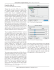### THREE DIMENSIONAL SYSTEMS Lecture 6: The Lorenz Equations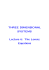### R - imeko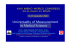### this product is designed for use in closed course racing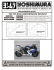### Intelligence in Living Cells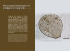### Lapidus Bunionectomy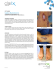### Lorenz system II - Harvard Mathematics Department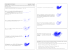### Troubleshooting Slow or No Treadle Movement### THIS PRODUCT IS DESIGNED FOR USE IN CLOSED### The 1960s: Pop, Op, and Minimalism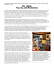### Spectrum of imaging features of SAPHO/ CRMO syndrome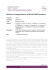### Acta Universitatis Lodziensis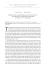### Vadim S. Anishchenko. - Dynamics, Bifurcations, and Strange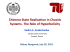### dissipative and weakly–dissipative regimes in nearly–integrable